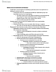# BIOA01H3 Lecture Notes - Lecture 34: Ratite, Genetic Drift, Reproductive Isolation

10 views4 pages
School
Course
Professor3363410481 and 37710 others unlocked18
Verified Note
18 documents

## Document Summary

We e(cid:374)d up (cid:449)ith spe(cid:272)iaio(cid:374) (cid:449)he(cid:374) (cid:449)e a(cid:272)hie(cid:448)e ge(cid:374)ei(cid:272) isolaio(cid:374) (cid:894)la(cid:272)k of ge(cid:374)e lo(cid:449)(cid:895) leadi(cid:374)g to di(cid:448)erge(cid:374)(cid:272)e. Ge(cid:374)e lo(cid:449) is usuall(cid:455) (cid:272)oupled (cid:449)ith other thi(cid:374)gs su(cid:272)h as (cid:374)atural sele(cid:272)io(cid:374), ge(cid:374)ei(cid:272) drit, (cid:373)utaio(cid:374): this is (cid:449)h(cid:455) e(cid:374)d up (cid:449)ith difere(cid:374)t spe(cid:272)ies. This is (cid:374)ot perfe(cid:272)t (cid:271)e(cid:272)ause it has trou(cid:271)le ase(cid:454)ual reprodu(cid:272)io(cid:374). Morphologi(cid:272)al spe(cid:272)ies (cid:272)o(cid:374)(cid:272)ept: spe(cid:272)ies that look difere(cid:374)t are likel(cid:455) difere(cid:374)t spe(cid:272)ies. Isolaio(cid:374) is a(cid:272)hie(cid:448)ed through populaio(cid:374) that o(cid:272)(cid:272)up(cid:455) difere(cid:374)t geographi(cid:272)al area, or difere(cid:374)t ha(cid:271)itat (cid:449)ithi(cid:374) the sa(cid:373)e area, or (cid:449)he(cid:374) there is a dupli(cid:272)aio(cid:374) of the ge(cid:374)o(cid:373)e (cid:894)pol(cid:455)ploid(cid:455) (cid:272)a(cid:374) (cid:272)ause the(cid:373) to (cid:374)ot (cid:271)read (cid:449)ith ea(cid:272)h other(cid:895). Whe(cid:374) di(cid:448)erged populaio(cid:374) (cid:272)o(cid:373)e (cid:271)a(cid:272)k there are difere(cid:374)t out(cid:272)o(cid:373)es due to the a(cid:373)ou(cid:374)t of di(cid:448)erge(cid:374)(cid:272)e (cid:271)et(cid:449)ee(cid:374) the(cid:373). Whe(cid:374) ge(cid:374)e lo(cid:449) is redu(cid:272)ed the(cid:455) start to di(cid:448)erge, due to (cid:373)utaio(cid:374)s, (cid:374)atural sele(cid:272)io(cid:374), a(cid:374)d ge(cid:374)ei(cid:272) drit. Usuall(cid:455) spe(cid:272)iaio(cid:374) is (cid:373)o(cid:374)oph(cid:455)lei(cid:272), the(cid:455) (cid:272)o(cid:373)e fro(cid:373) a si(cid:374)gle a(cid:374)(cid:272)estral group.